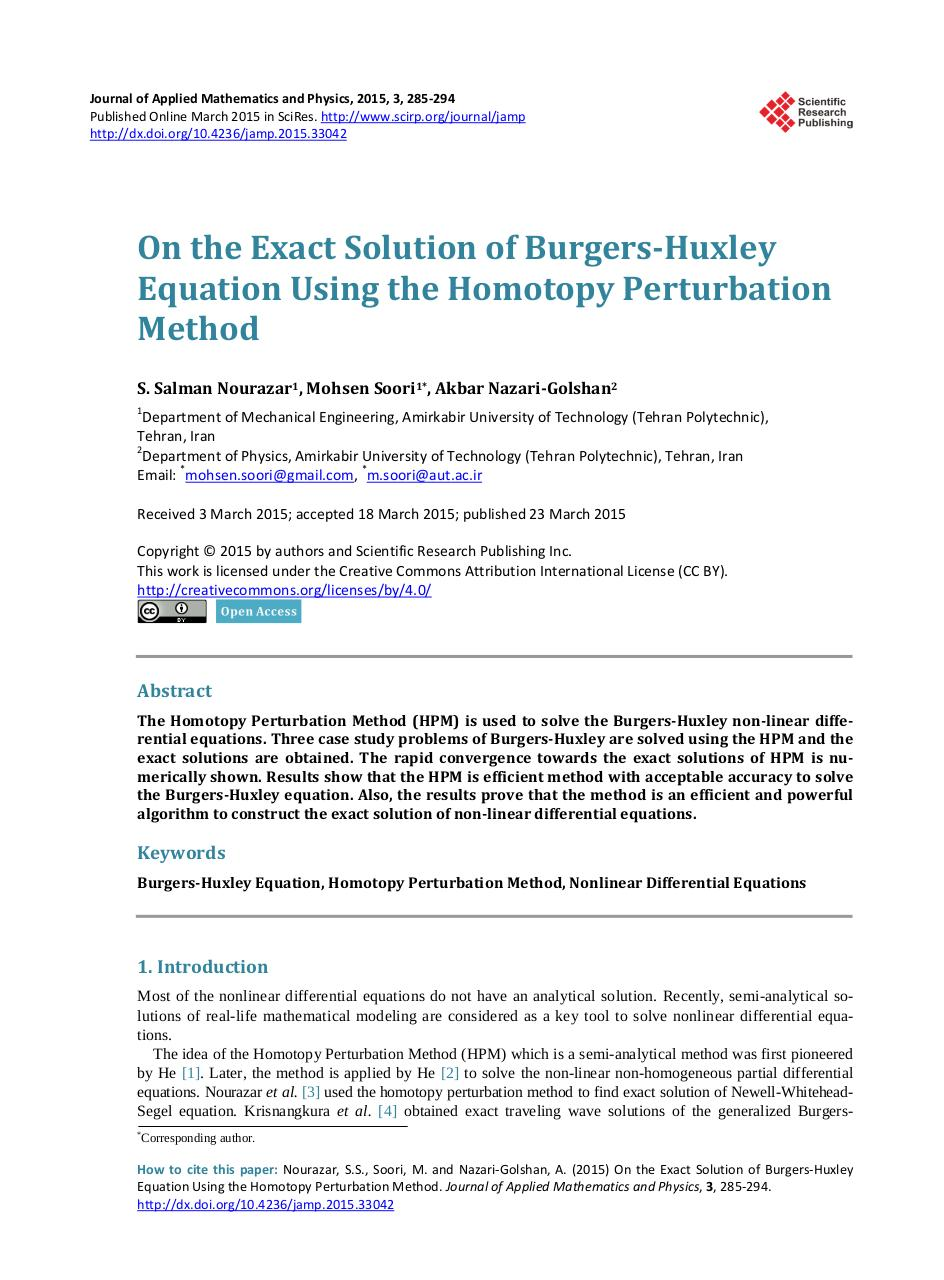# 285 294.pdfPage 1 2 3 4 5 6 7 8 9 10

#### Text preview

Journal of Applied Mathematics and Physics, 2015, 3, 285-294
Published Online March 2015 in SciRes. http://www.scirp.org/journal/jamp
http://dx.doi.org/10.4236/jamp.2015.33042

On the Exact Solution of Burgers-Huxley
Equation Using the Homotopy Perturbation
Method
S. Salman Nourazar1, Mohsen Soori1*, Akbar Nazari-Golshan2
1

Department of Mechanical Engineering, Amirkabir University of Technology (Tehran Polytechnic),
Tehran, Iran
2
Department of Physics, Amirkabir University of Technology (Tehran Polytechnic), Tehran, Iran
*
*
Email: mohsen.soori@gmail.com, m.soori@aut.ac.ir
Received 3 March 2015; accepted 18 March 2015; published 23 March 2015

Abstract
The Homotopy Perturbation Method (HPM) is used to solve the Burgers-Huxley non-linear differential equations. Three case study problems of Burgers-Huxley are solved using the HPM and the
exact solutions are obtained. The rapid convergence towards the exact solutions of HPM is numerically shown. Results show that the HPM is efficient method with acceptable accuracy to solve
the Burgers-Huxley equation. Also, the results prove that the method is an efficient and powerful
algorithm to construct the exact solution of non-linear differential equations.

Keywords
Burgers-Huxley Equation, Homotopy Perturbation Method, Nonlinear Differential Equations

1. Introduction
Most of the nonlinear differential equations do not have an analytical solution. Recently, semi-analytical solutions of real-life mathematical modeling are considered as a key tool to solve nonlinear differential equations.
The idea of the Homotopy Perturbation Method (HPM) which is a semi-analytical method was first pioneered
by He . Later, the method is applied by He  to solve the non-linear non-homogeneous partial differential
equations. Nourazar et al.  used the homotopy perturbation method to find exact solution of Newell-WhiteheadSegel equation. Krisnangkura et al.  obtained exact traveling wave solutions of the generalized Burgers*

Corresponding author.

How to cite this paper: Nourazar, S.S., Soori, M. and Nazari-Golshan, A. (2015) On the Exact Solution of Burgers-Huxley
Equation Using the Homotopy Perturbation Method. Journal of Applied Mathematics and Physics, 3, 285-294.
http://dx.doi.org/10.4236/jamp.2015.33042